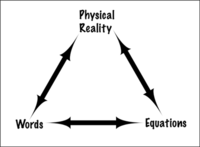# Teaching With Equations

What does that equation mean?
Jennifer Wenner, University of Wisconsin-Oshkosh; David Kohlstedt, University of Minnesota, Twin Cities; Anthony Hansen, St. Cloud State University in conjunction with the 2004 workshop.

## Increase students' comfort with equations

In upper level courses, geoscience students encounter equations with increasing frequency. Some may find the use or manipulation of equations to be challenging. These pages are designed to provide tips for helping students become comfortable with equations.

## Help students make connectionsTeaching students to make connections between physical world, words and equations is a fundamental skill for geoscientists. Physical reality, word descriptions and mathematical equations are all interconnected. Helping students realize these connections will build their confidence for using mathematics in a meaningful way.

## Use multiple representations

Students gain power in understanding equations when they see them expressed in different ways. Often students have some intuition about the behavior of the physical world because they have made many observations; however, they have difficulty connecting their intuition to mathematical expression. Giving students different ways of thinking about math and the physical world (with some guidance in the beginning) will empower them to connect the concepts on their own. Some of the tools that may be useful include: